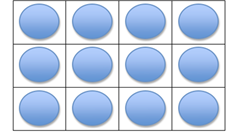Mathematics
Easy

Question

# Choose the multiplication expressions that match this array.## 4 × 2    4 × 4    3 × 4    3 × 3Hint:

## The correct answer is: 3 × 4

### In the question there is an array using which we have to find the multiplication expression of the given array.The total number of rows = 3The total number of columns = 4The multiplication expression= 3×4.So, the multiplication expression of the given array is 3×4.Therefore, the correct is c, i.e., 3×4.

For simple multiplication we have to remember the multiplication table of one-digit numbers and for two-digit number we can find it using the multiplication table of one-digit numbers. Here, we have to find the product of one digit number.

### Related Questions to study#### With Turito Foundation.#### Get an Expert Advice From Turito.